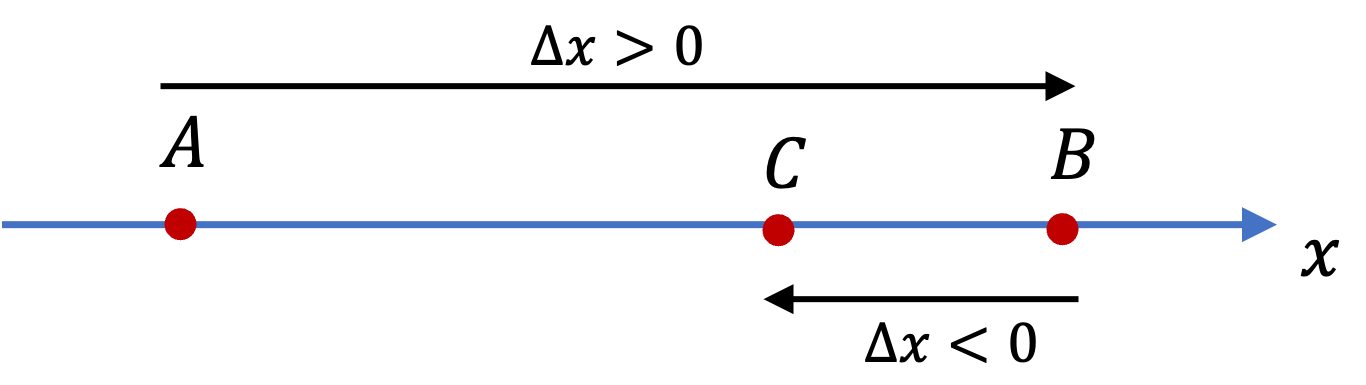## Section2.3Position, Displacement, and Distance

Position refers to the location of the object. For a motion occuring on a straight path, we will usually use $x$ axis to coincide with the path. In that case $x$ coordinate of the object will be its position. For vertical motions, we often use $y$ coordinate for the up and down straight path; in that case, it is the $y$ coordinate that will give position.

Most of our examples will be motion in a plane, either horizontal plane, verical plane, or slanted plane. For these types of motion, it is customary to use $xy$-plane for the plane. We, then use either the Cartesina coordinates $x\text{,}$ $y$ or polar coordinates, $r \text{,}$ $\theta$ for the motion. Polar coordinates are particularly useful when the motion is on a circle.

For arbitrary motion in a three-dimensional space we can use all Cartesian coordinates, $x,\ y,\ z \text{,}$ for the position. Other coordinate systems, such as spherical and cylindrical coordinates, are also used in particular situations. We probably will not have many examples of three-dimensional motion.

Displacement is the change in position during an interval. Displacements along coordinates are denoted by $\Delta x, \Delta y,$ and $\Delta z\text{.}$

Suppose, an object moves on a straight line. With $x$ axis along the motion, let position at initial instant $t_i$ be $x = x_i$ and at final instant $t_f$ be $x = x_f$ . Then, displacement during the interval $t_i$ to $t_f$ to will be

\begin{equation} \Delta x = x_f - x_i.\tag{2.3.1} \end{equation}

Clearly, if $x_f \gt x_i\text{,}$ displacement will be positive and if $x_f \lt x_i\text{,}$ it will be negative. Therefore, the sign of $\Delta x$ has the information about the direction of motion on the $x$ axis: positive means motion towards the $x=+\infty\text{,}$ negative means towards $x=-\infty\text{.}$Figure 2.3.1. A to B has $\Delta x \gt 0$ and B to C has $\Delta x \lt 0\text{.}$

Distance traveled during an interval, to be denoted by $d \text{,}$ refers to the length of the entire path of the motion, not just the initial and final locations. The distance is not the same thing as displacement. In Figure 2.3.1, the displacement of motion A-to-B-to-C is $x_C-x_A\text{,}$ but distance travelled is sum of the distances AB and BC.

\begin{equation*} d = \text{AB} + \text{BC},\ \ \ \text{but},\ \ \ \Delta x = x_\text{C} - x_\text{A}. \end{equation*}

As another example, consider going around a circle, returning to the same place. In this case, the displacment is zero because final position is same as the initial position, but the distance will be the circumference of the circle. The difference between displacement and distance is further illustrated in Checkpoint 2.3.2.

A boy runs on a straight track, which is taken to be the $x$ axis. As shown in Figure 2.3.3, the boy is at $x = 5\text{ m}$ mark at $t = 2 \text{ sec} \text{.}$ He runs to $x = 50\text{ m}$ mark, reaching there at $t=10\text{ sec}\text{.}$ He then turns around and runs back to $x = 20\text{ m}$ mark arriving there at $t = 20 \text{ sec}\text{.}$

(a) What is his displacement during the $t = 2 \text{ sec}$ to $t = 20 \text{ sec}$ interval?

(b) How much distance did he travel during the $t = 2 \text{ sec}$ to $t = 20 \text{ sec}$ interval?

(c) What is his displacement during the $t = 2 \text{ sec}$ to $t = 10 \text{ sec}$ interval?

(d) How much distance did he travel during the $t = 2 \text{ sec}$ to $t = 10 \text{ sec}$ interval?

(e) What is his displacement during the $t = 10 \text{ sec}$ to $t = 20 \text{ sec}$ interval?

(f) How much distance did he travel during the $t = 10 \text{ sec}$ to $t = 20 \text{ sec}$ interval?

Hint

Displacement depends only on the initial and final locations and distance depends on the entirety of motion.

(a) $\Delta x = 15\text{ m}\text{,}$ (b) $d = 75\text{ m}\text{,}$ (c) $\Delta x = 45\text{ m}\text{,}$ (d) $d = 45\text{ m}\text{,}$ (e) $\Delta x = -30\text{ m}\text{,}$ (f) $d = 30\text{ m}\text{,}$

Solution

(a) The displacement on $x$ axis is the change in the $x$ coordinates.

\begin{equation*} \Delta x = 20\text{ m} - 5\text{ m} = 15\text{ m}. \end{equation*}

(b) The distance traveled on path must take into account all the distance traveled. Here, the boy first ran $50-5 = 45 \text{ m}$ and then ran another $50 - 20 = 30\text{ m}$ on the return path. We need to add them.

\begin{equation*} d = 45 \text{ m} + 30\text{ m} = 75\text{ m}. \end{equation*}

(c)The displacement on $x$ axis is the change in the $x$ coordinates.

\begin{equation*} \Delta x = 50\text{ m} - 5\text{ m} = 45\text{ m}. \end{equation*}

(d) Since there is no turn in motion direction during this interval, the distance is equal to the magnitude of displacement.

\begin{equation*} d = 45\text{ m}. \end{equation*}

(e) The displacement on $x$ axis is the change in the $x$ coordinates.

\begin{equation*} \Delta x = 20\text{ m} - 50\text{ m} = -30\text{ m}. \end{equation*}

(f) Since there is no turn in motion direction during this interval, the distance is equal to the magnitude of displacement, stripping away the negative sign.

\begin{equation*} d = 30\text{ m}. \end{equation*}

A bottle rocket (firework) is shot vertically up from a platform (point A) that is $10\text{ m}$ above ground. The rocket flies to a height of $50\text{ m}$ (point B) before turning back and falling to the ground (point C). Use $y$ axis for displacements. What are the displacements and distances tavelled for the following motions: (a) A to B, (b) B to C, and (c) A-to-B-toC?